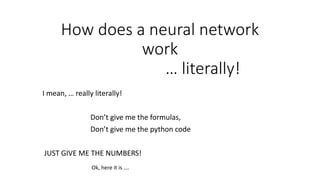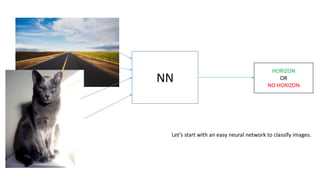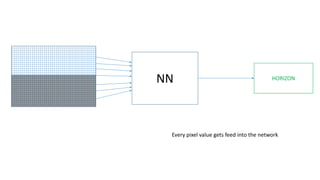Diese Präsentation wurde erfolgreich gemeldet.

# Neural network - how does it work - I mean... literally!×

1 von 33
1 von 33

# Neural network - how does it work - I mean... literally!

simple examples to understand neural network basics.
If you want to learn about neural networks you mostly see just creeeepy math formulas and symbols. In this presentation I leave all the creepy stuff out.
I just DO the math and the math is actually pretty easy - it's mostly just multiplying and adding numbers. But it's a lot of numbers! That's why it looks so complicated in formulas.

simple examples to understand neural network basics.
If you want to learn about neural networks you mostly see just creeeepy math formulas and symbols. In this presentation I leave all the creepy stuff out.
I just DO the math and the math is actually pretty easy - it's mostly just multiplying and adding numbers. But it's a lot of numbers! That's why it looks so complicated in formulas.

### Neural network - how does it work - I mean... literally!

1. 1. How does a neural network work … literally! I mean, … really literally! Don’t give me the formulas, Don’t give me the python code JUST GIVE ME THE NUMBERS! Ok, here it is ….
2. 2. NN HORIZON OR NO HORIZON Let’s start with an easy neural network to classify images.
3. 3. NN HORIZON Every pixel value gets feed into the network
4. 4. HORIZON At Wikipedia you find images like these, which show, that Every pixel value is feed into every neuron. Looks complicated! But the math is actually not that hard. It’ just a lot of calculations! So let’s break it down.
5. 5. HORIZON 1 0 Let’s focus on images with two pixels only. (for now)
6. 6. HORIZON 1 0 And let’s break it down to only one neuron! (for now)
7. 7. 1 0 1 For every input we have a wanted outcome. here it is 1,0 -> 1 At first we do not know, what outcome the neural net will be calculating. ?
8. 8. 1 0 1 * -0.16 * 0.99 Random Weights To start with the calculations, we set some random weights for the different inputs. These can be any numbers, but mostly one starts in the range of -1 to 1. With these we calculate our first inputs to the neuron. ? = -0.16 = 0.00
9. 9. 1 0 1 * -0.16 * 0.99 Random Weights Then we add up the results for every weight. ? = -0.16 = 0.00 + -0.16
10. 10. 1 * -0.16 = -0.16 0 * 0.99 = 0.0 1 0 + -0.16 > 0,46 0 1 Then we do one “higher math” step (not really), and get out 0,46 (Actually we just put -0.16 as x into the sigmoid-function, which is explaind the next slide and might look complicated but really isn’t.) 0,46 rounds to 0 which which is wrong … (we wanted 1) But that’s all there is to get results from a neural network that’s all there is in a neuron!
11. 11. 1 * -0.16 = -0.16 0 * 0.99 = 0.0 1 0 + -0.16 > 0,46 0 1 Example Input: Output: -1000 0.0 -100 0.0 -10 0.00004 -5 0.0066 -1 0.2689 -0.75 0.3208 -0.25 0.4378 0.0 0.5 0.25 0.5621 0.75 0.6791 1 0.7310 5 0.9933 10 0.9999 100 1.0 1000 1.0 The “higher math”: With the sum of the weighted inputs (-0.16) -> we call the sigmoid-function(x) Whoohooo! But what it does, is actually pretty easy: It maps every input to a value between 0 and 1 (see examples left) When we put -0.16 into the function we get 0.46 as a result. If we round this value we get 0 as an outcome of our neuron. This is wrong! What to do? Change the weights!
12. 12. 1 * -0.16 = -0.16 0 * 0.99 = 0.0 1 0 + -0.16 > 0,46 0 0.546 * 1 = 0.546 0.546 * 0 = 0.0 * 1- 0,46 = 0,546 1 1 -0.16+-0.546 = 0.38 0.99 + 0.0 = 0,99 += += 0.16 -> 0.38 0.99 -> 0.99 New weights: To correct the weights, we… …calculate the output error (0.546) …calculate the error per weight and input …and add it to the old weight. That’s called “Backpropagation” (because it goes backwards)
13. 13. 1 * 0.38 = 0.38 0 * 0.99 = 0.0 + 0.38 > 0,59 1 1 0 AND THAT‘s IT! Well… basically it really is. It‘s all about getting input values to the right output values by adjusting the weights. But obviously neural nets have more than one neuron. And normally there is more than one input. Let‘s increase the input‘s first! Now we calculate again, and get the right output!
14. 14. 1 1 0 1 0 1 Let‘s assume there is another input [1,1] which we want to map to 0. Now we have: This has some influence on how we adjust the weights on the way back – have a look:
15. 15. 1 * -0.16 = -0.16 1 * -0.16 = -0.16 0 * 0.99 = 0.00 1 * 0.99 = 0.99 1 0 + -0.16 -> 0.460 0.83 -> 0.696 0 0.546 * 1 = 0.546 -0.696 * 1 = -0.696 0.546 * 0 = 0.0 -0.696 * 1 = -0.696 * 1- 0.460= 0.546 0- 0.696= -0.696 1 -0.16+ 0.546 -0.696 = -0.316 0.99+ 0.0 -0.696 = +0.293 += += 1 1 1 0 0 1 0.16 -> -0.316 0.99 -> +0.293 Round 1 Now it‘s 2 values to add here…: We do the same for both inputs All what changes is how we sum up the new weight
16. 16. -0.16 + (1 * -0.546 + 1 * -0.696) = -0.16 + 0.546 - 0.696 = -0.316 We can write this update regarding to the errors in one line for each input weight: 0.99 + (0 * -0.546 + 1 * -0.696) = 0.99 + 0.000 - 0.696 = 0.293 0.16 -> -0.316 0.99 -> +0.293 0.546 * 1 = 0.546 -0.696 * 1 = -0.696 0.546 * 0 = 0.0 -0.696 * 1 = -0.696 * 1- 0.460= 0.546 0- 0.696= -0.696 -0.16+ 0.546 -0.696 = -0.316 0.99+ 0.0 -0.696 = +0.293 += += (we will do this again later when we have many weights…)
17. 17. 1 * -0.316 = -0.316 1 * -0.316 = -0.316 0 * 0.293 = 0.00 1 * 0.293 = 0.293 1 0 + -0.316 -> 0,421 -0.022 -> 0,494 0 0.578 * 1 = 0.578 -0.494 * 1 = -0.494 0,578 * 0 = 0.00 -0,494 * 1 = -0.494 * 1- 0,421= 0,578 0- 0,494= -0,494 1 -0.316+ 0.578 -0.494 = -0.232 0.293+ 0.0 -0.494 = -0.200 += += 1 1 0 0 0 1 -0.316 -> -0.232 +0.293 -> -0.200 Round 2 Still not right. So we do another round.
18. 18. 1 * -0.232 = 0.232 1 * -0.232 = 0.232 0 * -0.200 = 0.00 1 * -0.200 = -0.200 1 0 + -0.232 -> 0,442 -0.432 -> 0,393 01 1 0 -0.232 -> -0.067 -0.200 -> -0.594… … -> 1.087 … -> -0.934 … -> 0.483 … -> 0,340 … -> 0.277 … -> -1.238 … -> 0.527 … -> 0,304 … -> 0.568 … -> 0,276 … -> 0.431 … -> -1.515 … -> 0.968 … -> 0.021 … -> 3.426 … -> -7.271 Round 4 Round 3 Round 5 Round 6 Round 100 ……………………………………………………………………………………………………………………………. 1 0 And another And another And another … Till it fits!
19. 19. weight 1 (started at -0.16) weight 2 (started at 0.99) sum of errors the error converges to 0.0 (hopefully :-)
20. 20. Ok, I let out oooone little thing, which we need later (with more neurons and more layers) We do not actually take the error-values directly to correct the weights, but a weighted error… Have a look at sig‘(x): The results are always between 0 and 1 If the NN is not sure if 1 or 0 is the right answer: max=0.5 > maps to 0.25 Sure if 1 or 0: min=0 or 1 > maps to ~ 0.0 multiply the error with simgoid derivate of the result: e.g.: result = 0.46, error = 1-0.46 = 0.54 sigmoid derivate of result : 0.248 value for correction: 0.546 * 0.248 = 0.134 This practice assures, we give those errors more importance where we are unsure about the answer. sig‘(x)= sig(x)*(1-sig(x)) sig(x)= 1 (1 + e )-x We used this to map results to 0 or 1: We use this to evaluate which errors are more important
21. 21. 1 * -0.16 = -0.16 0 * 0.99 = 0.0 1 0 + -0.16 > 0,46 0 0.134 * 1 = 0.134 0.134 * 0 = 0.0 * 1- 0,46 = 0.546 0.46 > 0.248 0.546 * 0.248 = 0.134 1 1 -0.16+ 0.134= -0.025 0.99 – 0.0 = -0,99 += += 0.16 -> -0.025 0.99 -> 0.99 Now the weights are corrected more cautious: 0.16 -> 0.38 0.99 -> 0.99 Corrected weights before: Basically only this number changes a bit
22. 22. 1 * -0.16 = -0.16 0 * 0.99 = 0.0 1 0 + -0.16 > 0,46 0 0.546 * 1 = 0.546 0.546 * 0 = 0.0 * 1- 0,46 = 0,546 1 1 -0.16+-0.546 = 0.38 0.99 + 0.0 = 0,99 += += 0.16 -> 0.38 0.99 -> 0.99 New weights: To correct the weights, we… …calculate the output error (0.546) …calculate the error per weight and input …and add it to the old weight. That’s called “Backpropagation” (because it goes backwards) (s. slide 12)
23. 23. This was fun – let’s try another more complicated example with our neuron …. XOR
24. 24. 0 0 0 0 1 1 1 0 1 1 1 0 in out 0 0 0 0 1 1 1 0 1 1 1 0 XOR spoiler: does not work... (with one neuron)
25. 25. sum of error == 0! But…: [0,0] -> out = 0.5 -> error = -0.5 [0,1] -> out = 0.5 -> error = 0.5 [1,0] -> out = 0.5 -> error = 0.5 [1,1] -> out = 0.5 -> error = -0.5 The weights are actually both 0! If you think about it, it‘s pretty obvious. Every weight has to be the same, because a 1 or 0 leads to 0 or 1 in the same amount of cases For XOR to work we need more layers!
26. 26. 0 1 1 ? We try it with three input neurons and one output neuron Let’s look at only one input first.
27. 27. 0 1 0.81 -0.84 0.299 0.90 -0.63 0.588 -0.77 0.88 0.707 -0.79 0.69 0.667 0.80 sig( 0*0.81 + 1*-0.84 ) = sig(-0.84) = 0.299 sig( 0.299*0.90 + 0.707*-0.63 + 0.667*0,80) = sig(-0.353) = 0.588 The forward calculation for one input The output is 1 that’s good! – let’s check the other inputs: layer0 layer1 In layer2 we have not 0 or 1 as input, but fractions! 1 !
28. 28. layer2layer0 layer1 0.81 -0.84 0.500 0.299 0.692 0.491 0.90 -0.63 0.630 0.588 0.662 0.619 -0.77 0.88 -0.79 0.69 0.80 0.500 0.707 0.315 0.527 0.500 0.667 0.310 0.474 first results for all inputs (not so good…) The forward calculation for ALL FOUR inputs 1.0 1.0 1.0 1.0 For all 4 inputs the results are not so good… we have to update the weights… layer by layer…
29. 29. 0.81 -0.84 0.84 -0.69-0.77 0.88 -0.79 0.69 0.75 l2_error 0-r1= -0.630 1-r2= 0.411 1-r3= 0.337 0-r4= -0.619 l2_delta sig‘(0.63)*-0.63=-0.146 sig‘(0.58)* 0.41= 0.099 sig‘(0.66)* 0.34= 0.075 sig‘(0.62)*-0.62=-0.14 0.90 + (0.500*-0.146 + 0.299*0.099 + 0.692*0.075 + 0.491*-0.14) = 0.84 -0.63 + (0.500*-0.146 + 0.707*0.099 + 0.315*0.075 + 0.527*-0.14) = -0.69 0.80 + (0.500*-0.146 + 0.667*0.099 + 0.310*0.075 + 0.474*-0.14) = 0.75 same as before (but with more values…) 0.500 0.299 0.692 0.491 0.500 0.707 0.315 0.527 0.500 0.667 0.310 0.474 * * * * += += += += += += 0,0 -> r1= 0.630 0,1 -> r2= 0.588 1,0 -> r3= 0.662 1,1 -> r4= 0.619 Backward Propagation: Now we update the old weights regarding to the errors of all inputs. First in layer 2 0.90 -0.63 0.80
30. 30. 0.795 -0.861 0.81 + (0*-0.033 + 0*0.018 + 1*0.014 + 1*-0.033) = 0.795 -0.84 + (0*-0.033 + 1*0.018 + 0*0.014 + 1*-0.033) = -0.861 Again as before (but with more values…) 0.500 0.299 0.692 0.491 * * * += += += += 0,0 -> r1= 0.5 0,1 -> r2= 0.299 1,0 -> r3= 0.692 1,1 -> r4= 0.491 l1_delta sig‘(0.500) *-0.132 =-0.033 sig‘(0.299) * 0.090 = 0.018 sig‘(0.692) * 0.068 = 0.014 sig‘(0.491) *-0.131 =-0.033 l1_error = l2_delta * weight of neuron 1 (to l2) 0,0 -> -0.146*0.90 = -0.132 0,1 -> 0.099*0.90 = 0.090 1,0 -> 0.075*0.90 = 0.068 1,1 -> 0.140*0.90 = -0.131 And now the weights in layer 1! The error in neuron1 is a fraction of the error in the output neuron (l2) Therefore we take the delta-error of layer2; neuron1 accounted 0.90 to this error: l2_delta * weight of neuron 1 to l2 And of this we get the delta again -> l1_delta * += += 0.81 -0.84 Neuron 1 in layer 1:
31. 31. error curve (depends strongly on the start weights) np.random.seed(11) np.random.seed(44) np.random.seed(101) np.random.seed(1023) but in converges pretty good (mostly…) Others: (for python users: the starter weights have been generated with: np.random.seed(245))
32. 32. np.random.seed(25) WOOPS! Output: 0,0 -> [ 0.0214737 ] 0,1 -> [ 0.98097769] 1,0 -> [ 0.9810124 ] 1,1 -> [ 0.50051869] ? – probably runs into a local minimum…
33. 33. That’s it so far – hope you had a good ride. Obviously there are more questions coming up after this session, which I might dig into later: • Why are there so different error curves? • How to avoid a wrong output like the last error curve. • How many iterations does a neural network has to do, to learn? • How many neurons to take? • How deep the layers should be (deep learning)? • Are there other functions to use instead of the sigmoid function? • Different nets working together • etc etc…